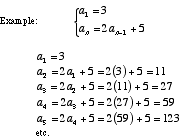index: click on a letter A B C D E F G H I J K L M N O P Q R S T U V W X Y Z A to Z index index: subject areas numbers & symbols sets, logic, proofs geometry algebra trigonometry advanced algebra & pre-calculus calculus advanced topics probability & statistics real world applications multimedia entrieswww.mathwords.com about mathwords website feedback

 Recursive Formula For a sequence a1, a2, a3, . . . , an, . . . a recursive formula is a formula that requires the computation of all previous terms in order to find the value of an . Note: Recursion is an example of an iterative procedure.See also Explicit formula